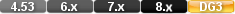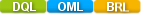Easy to Create, Easy to Change - Easy to use!## Asin (arcsine)asin(numeric field) asin(numeric value) asin(-1) asin(0.5)

The asin function calculates the arcsine of a numeric value. The result is an angle expressed in radians between -p/2 to p/2.

# Parameters

Numerical value between -1 via 0 to +1

A numeric value

# Examples

Example 1

asin(1)

Result:1.570796

Example 2

asin(-0.50)

Result: -0.5235988

# asin (arcsine)

#### Type

Trigonometric Function

#### Purpose

The asin function calculates the arcsine of a numeric value. The result is an angle expressed in radians between -p/2 to p/2.

Syntax

asin( NUMERIC VALUE)

#### Returns

A numeric value.

Usage

The numeric value in a Trigonometric function can be a constant value (as shown below), a variable, a field value or an expression.

The valid range of the input value is -1 to 1.

#### Example

asin(1)

Returns:1.570796

asin(-0.50)

Returns: -0.5235988